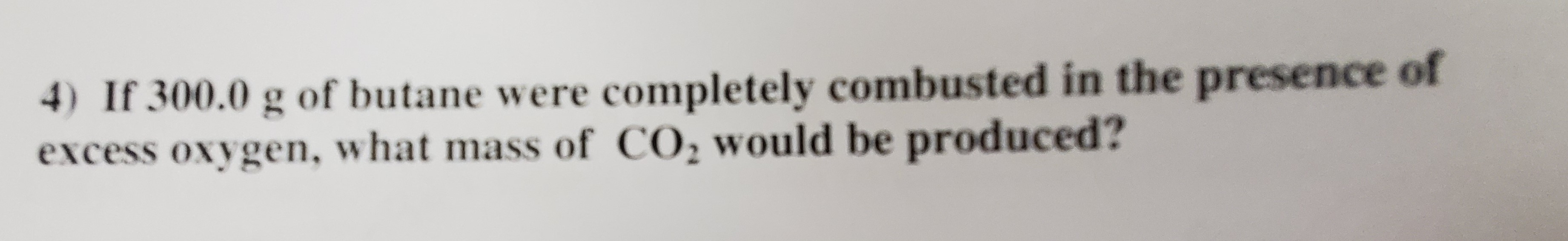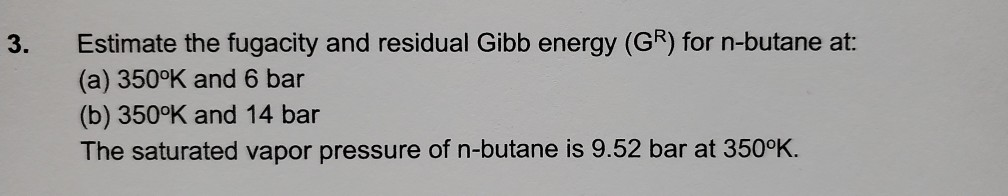Question

# What energy results when "n-butane" is combusted?

What energy results when "n-butane" is combusted?

Answer 1

And where are the data...?

#### Explanation:

You want the enthalpy associated with the reaction...

${C}_{4} {H}_{10} \left(g\right) + \frac{13}{2} {O}_{2} \left(g\right) \rightarrow 4 C {O}_{2} \left(g\right) + 5 {H}_{2} O \left(l\right) + \Delta$

This site quotes for butane, $\Delta {H}_{\text{combustion}}^{\circ} = - 2.88 \times {10}^{3} \cdot k J \cdot m o {l}^{-} 1$...in this instance $k J \cdot m o {l}^{-} 1$ means PER MOLE of reaction as written.

#### Earn Coins

Coins can be redeemed for fabulous gifts.

Similar Homework Help Questions
• ### 4) If 300.0 g of butane were completely combusted in the presence of excess oxygen, what...4) If 300.0 g of butane were completely combusted in the presence of excess oxygen, what mass of CO2 would be produced?

• ### How much energy will be released to the surroundings when 0.100 g of ethanol are combusted?...

How much energy will be released to the surroundings when 0.100 g of ethanol are combusted? CH3CH2OH (l) + 302 (g) → 2C02 (g) +3H2O (g) deltaHrxn = -1235 k.J

• ### How much energy is released when 14.0 g of carbon monoxide are completely combusted?

2CO_((g)) + O_(2(g)) -> 2CO_(2(g))" " DeltaH = -"560 kJ"#

• ### 1. 5.00 g of butane are combusted in a sealed container containing 2.00 g of oxygen...

1. 5.00 g of butane are combusted in a sealed container containing 2.00 g of oxygen gas. The balanced reaction for the combustion of butane is shown below. How many grams of the excess reagent are left over? (This question first requires you to determine the limiting reagent, and then calculate how much will be leftover). Pease answer in g. Molar Mass Butane: 58.12 g/mol Molar Mass Oxygen Gas: 32.0 g/mol 2CH3CH2CH2CH3 + 13O2 --> 8CO2 + 10H2O 2. If...

• ### n-butane and isobutane are in equilibrium at 25 degrees centigrade:    n-butane (g)n-butane and isobutane are in equilibrium at 25 degrees centigrade:    n-butane (g)                           isobutane (g)           A one liter flask with the two species at equilibrium contains 0.170 mole isobutane and 0.0680 mole n-butane. Then the concentration of n-butane is increase by 0.200 moles. (0.200 mole n-butane is added.) What is the new equilibrium concentration for isobutane? Assume value for K c remains constant throughout process. Hint--this is a hard problem. At equilibrium (which you are given) you can find...

• ### Estimate the fugacity and residual Gibb energy (GR) for n-butane (30) at: (a) 350oK and 4...

Estimate the fugacity and residual Gibb energy (GR) for n-butane (30) at: (a) 350oK and 4 bar (b) 350oK and 25 bar

• ### 3. Estimate the fugacity and residual Gibb energy (GR) for n-butane at: (a) 350°K and 6...3. Estimate the fugacity and residual Gibb energy (GR) for n-butane at: (a) 350°K and 6 bar (b) 350°K and 14 bar The saturated vapor pressure of n-butane is 9.52 bar at 3500K.